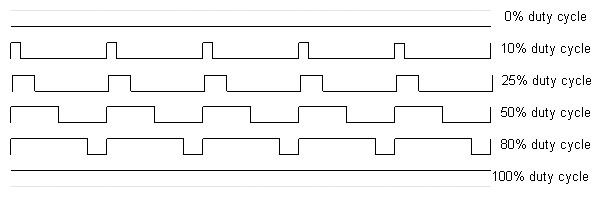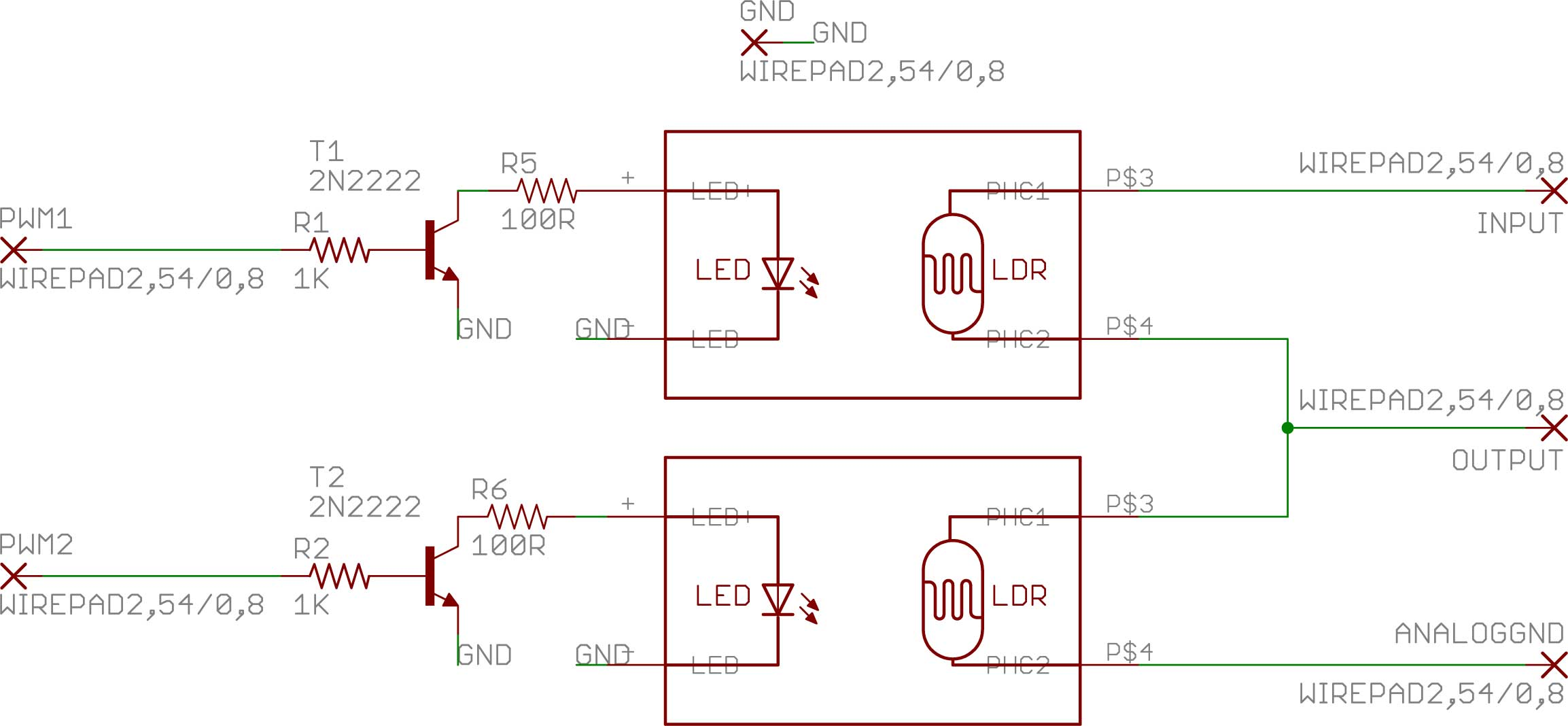# VC-1: Digital/Midi Volume Control

A user asked if I could design an FCB1010/PU-2 volume control. I love challenges…

It took me weeks to get one working properly. The are two main parts to this: the analog side and the digital side.

The analog side

A potentiometer will generally be connected between ground and signal as:In this case, the output impedance is determined by the value of the base resistance of the potentiometer and is considered constant on the output signal because the variable resistance of the pot is always mixing some ground and input signal. As an example, take a 5K pot: if the wiper (the movable part of the potentiometer) is all the way to the IN side, the output is equal to the input signal. The impedance is determined by the resistance to GROUND,  5K. If the wiper is on the other end of it’s travel, the output becomes = GROUND and no sound can be heard. The impedance is still 5K, as the resistance between the wiper and the IN signal is still determined by the pot resistance.

Now, if the wiper is placed somewhere between the two extreme, the output signal is a combination of IN and GROUND. Let’s say the wiper is 1/5th the way from IN. The resistance (if the pot is linear (that’s another story)) between IN and OUT is about 1K. The resistance between GROUND and OUT is about 4K. The impedance is (Resistance from IN to OUT) + (resistance from GROUND to out) = 5K.

That, in a simplified way, is how it works.

One might be tempted to use the pot in the following manner:

but this will not work, especially when the resistance decreases. It will create all sorts of distortion and the equipment in the rest of your audio chain will definitely not appreciate the lack of impedance control.

After quite a bit of research, I decided to use the Silonex Audiohm optocouplers (http://www.silonex.com/audiohm/index.html). A LED turns on and off depending on  the voltage present on its leads. It is closely coupled with a Light Detecting Resistor (LDR) and affects the resistance on the output side. The ones I chose (NSL-32SR3) have an “empty” resistance of 25M Ohms and go down to 40 Ohms when fully energized (about 5 volts).In theory, this is how the circuit should work. The signal is fed from the PU-2’s expression pedal to the micro-controller and a small piece of code writes to the PWM pins (Pulse Width Modulation) to control the LED on each of two LDRs. Why two? If you understood the beginning of this section, you will understand that the upper LDR will act as the half of the potentiometer that ties IN to OUT, and that the bottom LDR then acts as the half of the potentiometer that ties GROUND to OUT, to preserve the output impedance.

The trick here is to feed a proportional signal to the two LDRs so that their combined resistance is pretty much constant.

The signal that drives the LDRs is digital. So let’s take a look at that and we will come back to the analog side later.

The Digital Side

The signal that will drive the LDRs is coming from two digital pins on the Arduino. The pins have to provide PWM output. PWM is a simulation of analog voltage output. The microcontroller is providing this simulated voltage by sending pulses of voltage (5 volts) at controlled intervals. For example, if the microcontroller send 5 volts for 1/4 of a second and then sends 0 volts for 3/4 of a second, a component might believe that it’s receiving 1.25 volts (1/4 of 5 volts). Vaguely.There’s a decent tutorial on the Arduino site (http://www.arduino.cc/en/Tutorial/PWM) and a very complete one at http://en.wikipedia.org/wiki/Pulse-width_modulation. You can find many others with a simple Google.

Oddly enough, it doesn’t really work in practice. The LDRs are quick enough to react to the square wave signal and introduce artifacts (awful distortion!) in the audio signal. I figured that the LDRs would behave better if fed a true voltage. So I decided to insert an RC filter (http://en.wikipedia.org/wiki/RC_circuit) in front of the LDR to smooth things out. I couldn’t find one that worked…

Then, I figured that the signal could be made faster by making the PWM pulse faster. The logic was that if I could push the PWM frequency outside of the audio spectrum (above 20,000 Hz) I would eliminate the interference. It worked… partly.

This line of code:

`  TCCR0B = TCCR0B & 0b11111000 | 0x01; //adjust PWM frequency to put it ouside hearing range (62K)`

forces the Arduino PWM on certain pins to “vibrate” at 62500 Hz. While this is enough to get most of the distortion outside of the audio spectrum, it changes the behaviour of the LDRs. They loose their mind…

I tried other RC filters/PWM speeds combination to no avail. Nothing worked.

Finally, I suspected that there might be a current drop on the LED inside the LDR. The datasheet specifies 25mA maximum for the LED. But can the Arduino supply enough current when operating in PWM mode?

I decided to add a simple transistor in series with the PWM pin to augment the current to the LDR’s  LED. (How many 3-letter abbreviations can I put in a single sentence?) So I chose a 2N2222 transistor wired this way:This worked a lot better!

The Arduino code I used for testing:

This entry was posted in Arduino, FCB1010, Pedal board, PU-2, VC-1 and tagged . Bookmark the permalink.

### 3 Responses to VC-1: Digital/Midi Volume Control

1.Peter Sharp says:

Robert,
You said, “How many 3-letter abbreviations can I put in a single sentence?” – you obviously don’t work at IBM! Glad to hear you found a working solution 🙂

Peter

2.Andrew says:

The problem with the LDR circuit is the response times for the LDR devices.. what you need to do as add a solid state integrator, also known as a low pass filter, to convert the PWM to a stable DC voltage that is proportional to the off/on times of the PWM signal. A simple RC circuit would do to try it with, but a constant current integrator would give less noise, and better response.

•rt says:

Andrew,
I am using a low pass filter in the second version of the VC-1. See post http://practicalusage.com/?p=702

Robert.